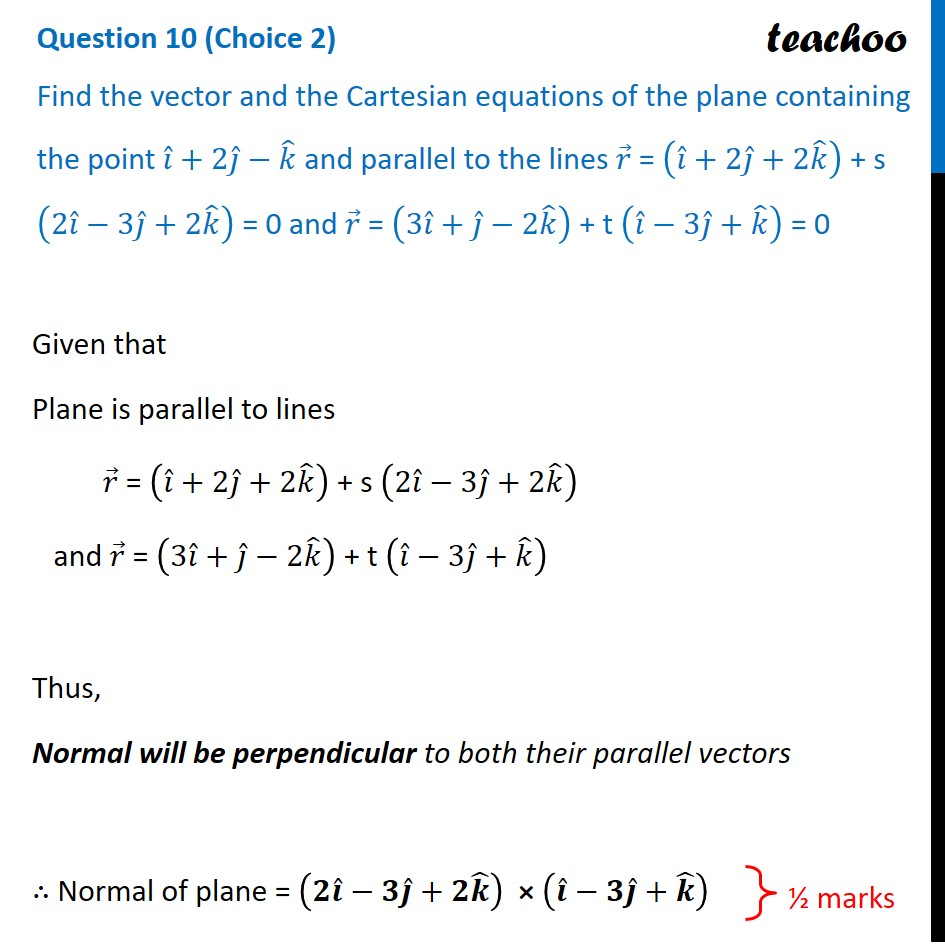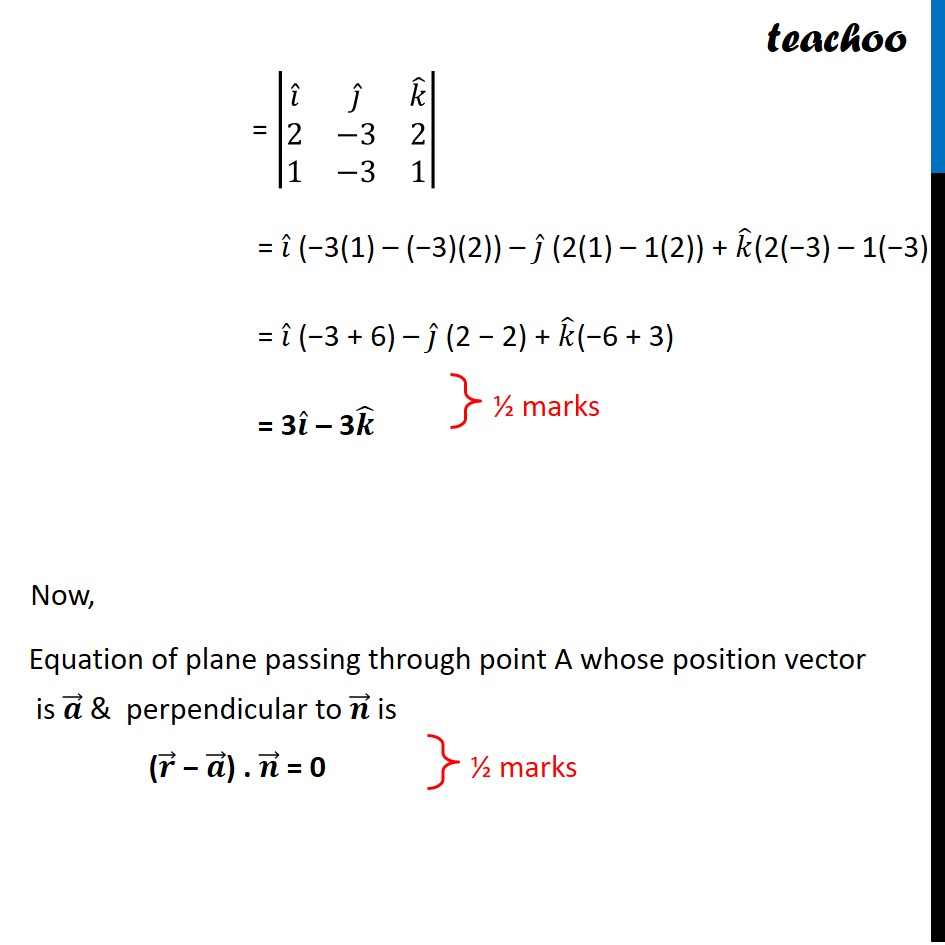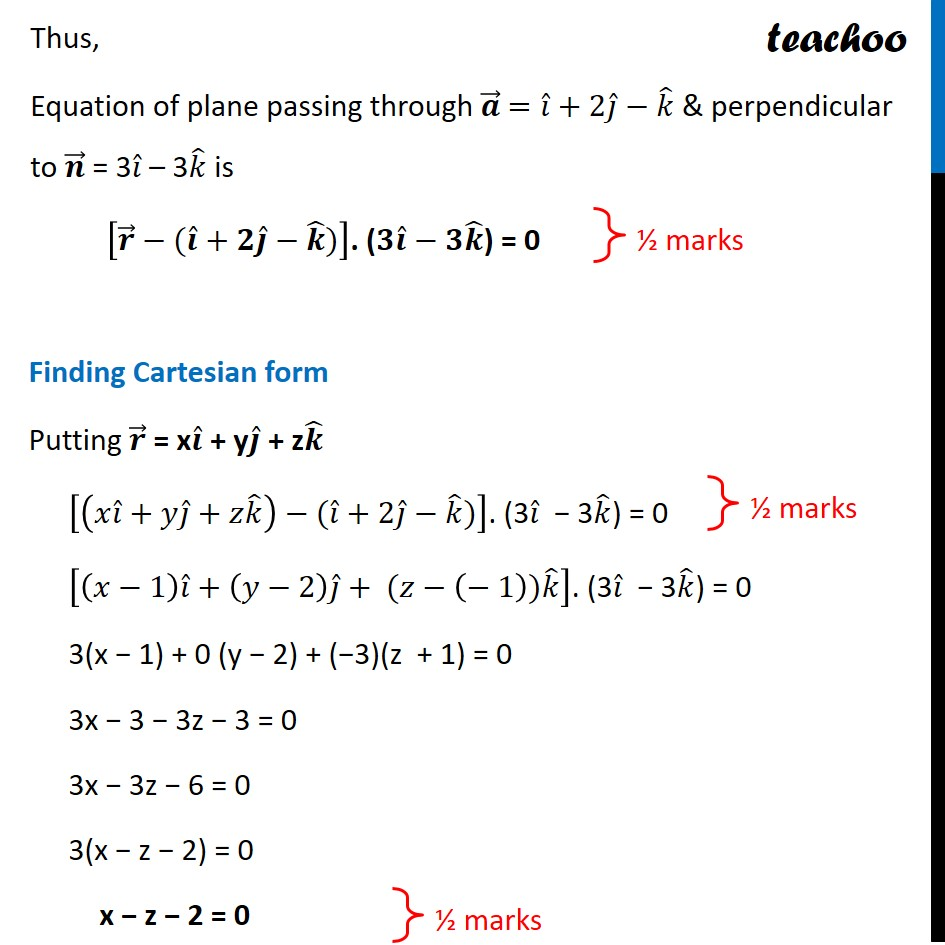CBSE Class 12 Sample Paper for 2022 Boards (For Term 2)

Class 12
Solutions of Sample Papers and Past Year Papers - for Class 12 Boards

## Find the vector and the Cartesian equations of the plane containing the point 𝑖 ̂+2𝑗 ̂−𝑘 ̂ and parallel to the lines 𝑟 ⃗ = (𝑖 ̂+2𝑗 ̂+2𝑘 ̂ ) + s (2𝑖 ̂−3𝑗 ̂+2𝑘 ̂ ) = 0 and 𝑟 ⃗ = (3𝑖 ̂+𝑗 ̂−2𝑘 ̂ ) + t (𝑖 ̂−3𝑗 ̂+𝑘 ̂ ) = 0

This question is similar to Misc 13 Chapter 11 Class 12 - Three Dimensional GeometryLearn in your speed, with individual attention - Teachoo Maths 1-on-1 Class

### Transcript

Question 10 (Choice 2) Find the vector and the Cartesian equations of the plane containing the point 𝑖 ̂+2𝑗 ̂−𝑘 ̂ and parallel to the lines 𝑟 ⃗ = (𝑖 ̂+2𝑗 ̂+2𝑘 ̂ ) + s (2𝑖 ̂−3𝑗 ̂+2𝑘 ̂ ) = 0 and 𝑟 ⃗ = (3𝑖 ̂+𝑗 ̂−2𝑘 ̂ ) + t (𝑖 ̂−3𝑗 ̂+𝑘 ̂ ) = 0 Given that Plane is parallel to lines 𝑟 ⃗ = (𝑖 ̂+2𝑗 ̂+2𝑘 ̂ ) + s (2𝑖 ̂−3𝑗 ̂+2𝑘 ̂ ) and 𝑟 ⃗ = (3𝑖 ̂+𝑗 ̂−2𝑘 ̂ ) + t (𝑖 ̂−3𝑗 ̂+𝑘 ̂ ) Thus, Normal will be perpendicular to both their parallel vectors ∴ Normal of plane = (𝟐𝒊 ̂−𝟑𝒋 ̂+𝟐𝒌 ̂ ) × (𝒊 ̂−𝟑𝒋 ̂+𝒌 ̂ ) = |■8(𝑖 ̂&𝑗 ̂&𝑘 ̂@2&−3&2@1&−3&1)| = 𝑖 ̂ (−3(1) – (−3)(2)) – 𝑗 ̂ (2(1) – 1(2)) + 𝑘 ̂(2(−3) – 1(−3)) = 𝑖 ̂ (−3 + 6) – 𝑗 ̂ (2 − 2) + 𝑘 ̂(−6 + 3) = 3𝒊 ̂ – 3𝒌 ̂ Now, Equation of plane passing through point A whose position vector is 𝒂 ⃗ & perpendicular to 𝒏 ⃗ is (𝒓 ⃗ − 𝒂 ⃗) . 𝒏 ⃗ = 0 Thus, Equation of plane passing through 𝒂 ⃗ =𝑖 ̂+2𝑗 ̂−𝑘 ̂ & perpendicular to 𝒏 ⃗ = 3𝑖 ̂ – 3𝑘 ̂ is [𝒓 ⃗−(𝒊 ̂+𝟐𝒋 ̂−𝒌 ̂)]. (𝟑𝒊 ̂−𝟑𝒌 ̂) = 0 Finding Cartesian form Putting 𝒓 ⃗ = x𝒊 ̂ + y𝒋 ̂ + z𝒌 ̂ [(𝑥𝑖 ̂+𝑦𝑗 ̂+𝑧𝑘 ̂ )−(𝑖 ̂+2𝑗 ̂−𝑘 ̂)]. (3𝑖 ̂ − 3𝑘 ̂) = 0 [(𝑥−1) 𝑖 ̂+(𝑦−2) 𝑗 ̂+ (𝑧−(− 1))𝑘 ̂ ]. (3𝑖 ̂ − 3𝑘 ̂) = 0 3(x − 1) + 0 (y − 2) + (−3)(z + 1) = 0 3x − 3 − 3z − 3 = 0 3x − 3z − 6 = 0 3(x − z − 2) = 0 x − z − 2 = 0